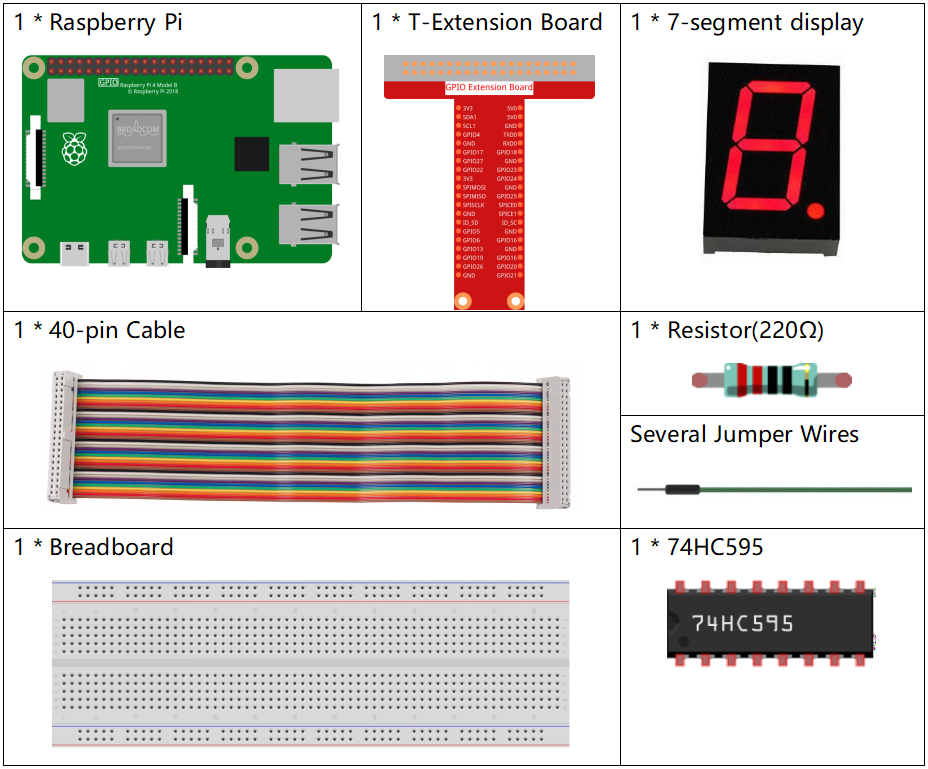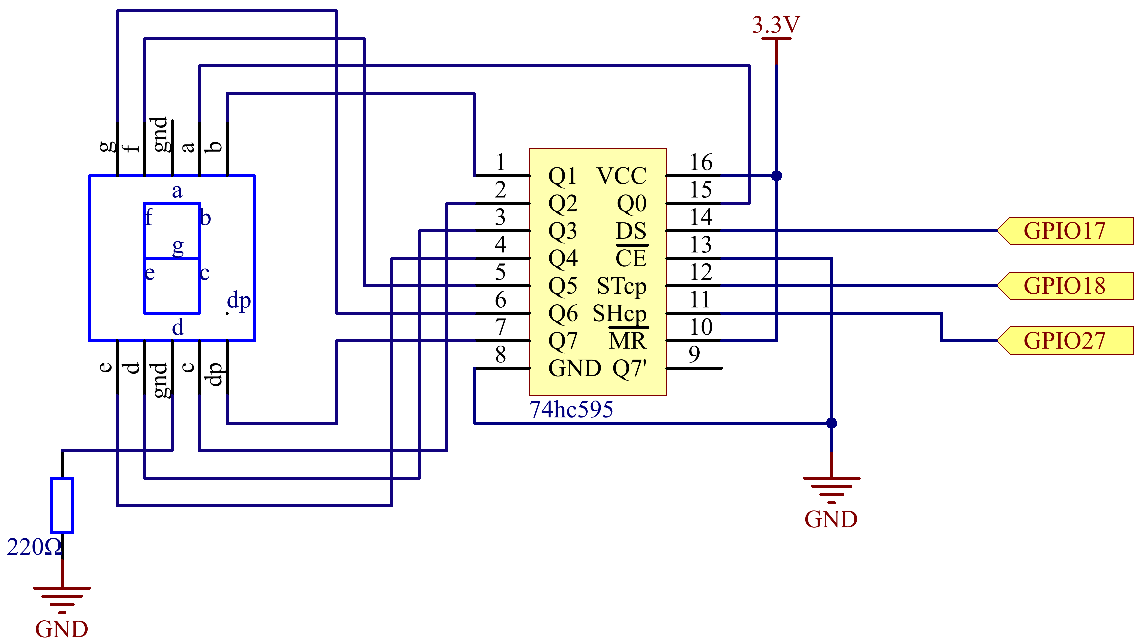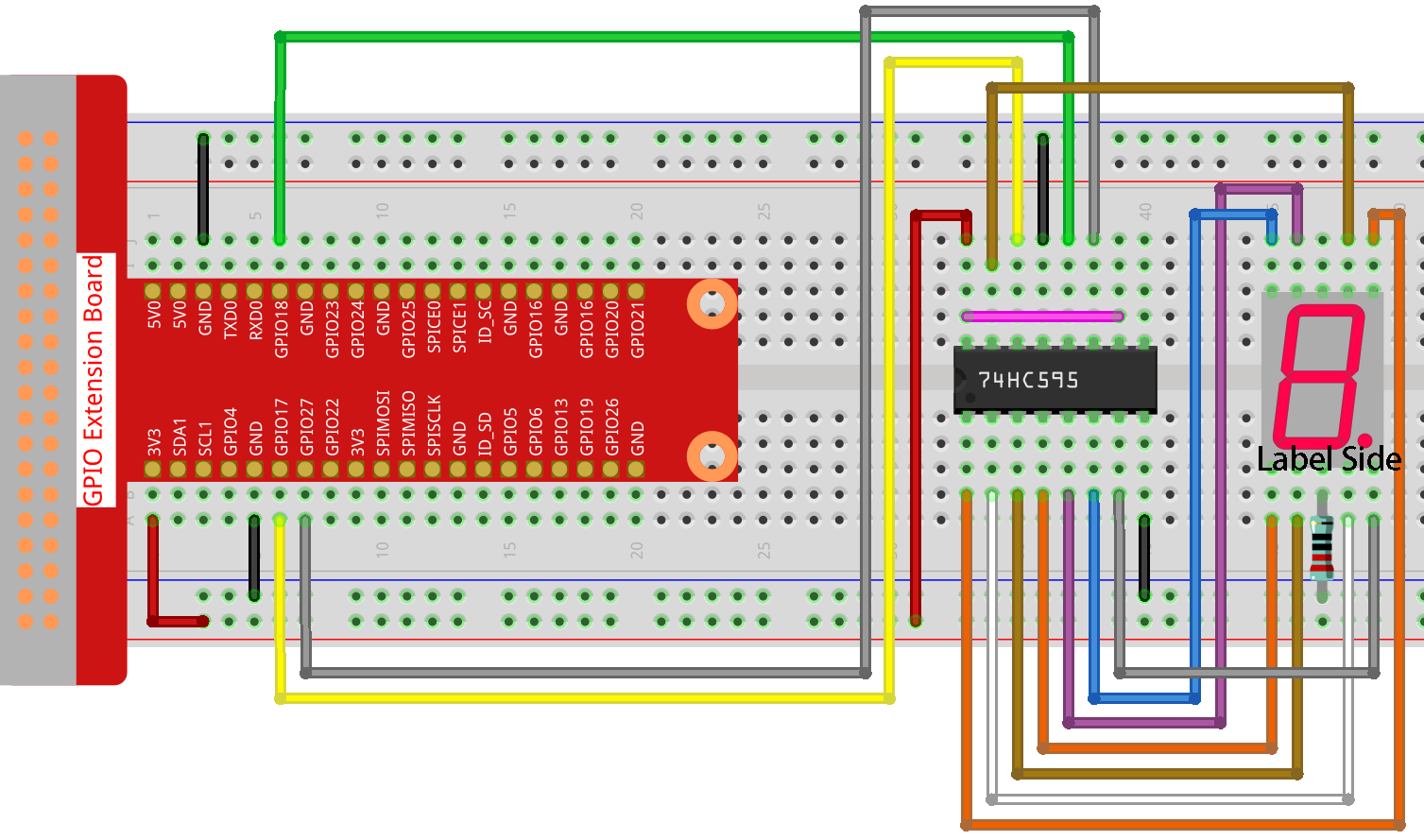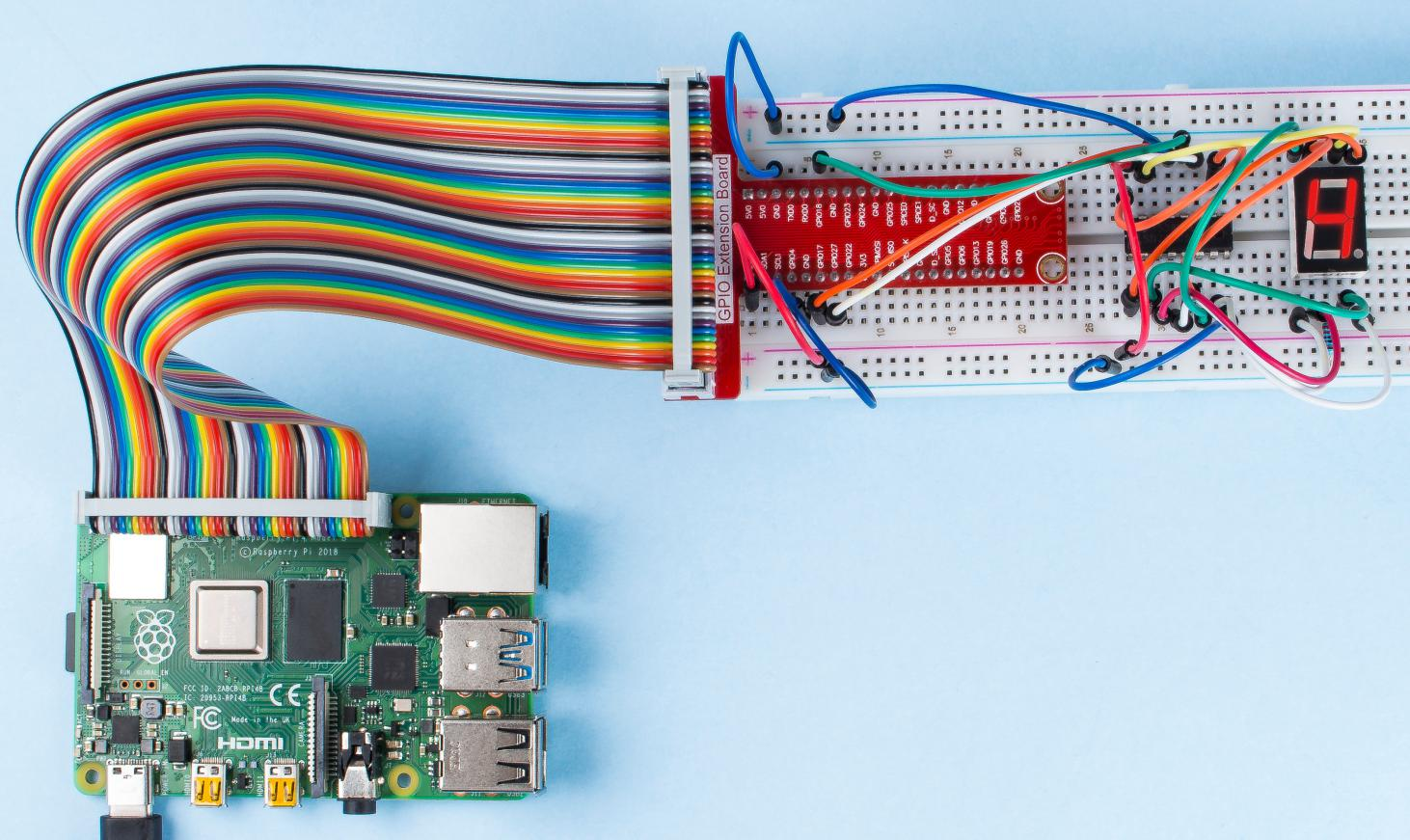# 1.1.4 7-segment Display¶

## Introduction¶

Let’s try to drive a 7-segment display to show a figure from 0 to 9 and A to F.

## Components¶## Schematic Diagram¶

Connect pin ST_CP of 74HC595 to Raspberry Pi GPIO18, SH_CP to GPIO27, DS to GPIO17, parallel output ports to 8 segments of the LED segment display. Input data in DS pin to shift register when SH_CP (the clock input of the shift register) is at the rising edge, and to the memory register when ST_CP (the clock input of the memory) is at the rising edge. Then you can control the states of SH_CP and ST_CP via the Raspberry Pi GPIOs to transform serial data input into parallel data output so as to save Raspberry Pi GPIOs and drive the display.

 T-Board Name physical wiringPi BCM GPIO17 Pin 11 0 17 GPIO18 Pin 12 1 18 GPIO27 Pin 13 2 27## Experimental Procedures¶

Step 1: Build the circuit.Step 2: Get into the folder of the code.

```cd /home/pi/raphael-kit/python/
```

Step 3: Run.

```sudo python3 1.1.4_7-Segment.py
```

After the code runs, you’ll see the 7-segment display display 0-9, A-F.

Code

Note

You can Modify/Reset/Copy/Run/Stop the code below. But before that, you need to go to source code path like `raphael-kit/python`. After modifying the code, you can run it directly to see the effect. After confirming that there are no problems, you can use the Copy button to copy the modified code, then open the source code in Terminal via `nano` cammand and paste it.

```import RPi.GPIO as GPIO
import time

# Set up pins
SDI   = 17
RCLK  = 18
SRCLK = 27

# Define a segment code from 0 to F in Hexadecimal
segCode = [0x3f,0x06,0x5b,0x4f,0x66,0x6d,0x7d,0x07,0x7f,0x6f,0x77,0x7c,0x39,0x5e,0x79,0x71]

def setup():
GPIO.setmode(GPIO.BCM)
GPIO.setup(SDI, GPIO.OUT, initial=GPIO.LOW)
GPIO.setup(RCLK, GPIO.OUT, initial=GPIO.LOW)
GPIO.setup(SRCLK, GPIO.OUT, initial=GPIO.LOW)

# Shift the data to 74HC595
def hc595_shift(dat):
for bit in range(0, 8):
GPIO.output(SDI, 0x80 & (dat << bit))
GPIO.output(SRCLK, GPIO.HIGH)
time.sleep(0.001)
GPIO.output(SRCLK, GPIO.LOW)
GPIO.output(RCLK, GPIO.HIGH)
time.sleep(0.001)
GPIO.output(RCLK, GPIO.LOW)

def main():
while True:
# Shift the code one by one from segCode list
for code in segCode:
hc595_shift(code)
print ("segCode[%s]: 0x%02X"%(segCode.index(code), code)) # %02X means double digit HEX to print
time.sleep(0.5)

def destroy():
GPIO.cleanup()

if __name__ == '__main__':
setup()
try:
main()
except KeyboardInterrupt:
destroy()
```

Code Explanation

```segCode = [0x3f,0x06,0x5b,0x4f,0x66,0x6d,0x7d,0x07,0x7f,0x6f,0x77,0x7c,0x39,0x5e,0x79,0x71]
```

A segment code array from 0 to F in Hexadecimal (Common cathode).

```def setup():
GPIO.setmode(GPIO.BCM)
GPIO.setup(SDI, GPIO.OUT, initial=GPIO.LOW)
GPIO.setup(RCLK, GPIO.OUT, initial=GPIO.LOW)
GPIO.setup(SRCLK, GPIO.OUT, initial=GPIO.LOW)
```

Set ds, st_cp, sh_cp three pins to output and the initial state as low level.

```GPIO.output(SDI, 0x80 & (dat << bit))
```

Assign the dat data to SDI(DS) by bits. Here we assume dat=0x3f(0011 1111, when bit=2, 0x3f will shift right(<<) 2 bits. 1111 1100 (0x3f << 2) & 1000 0000 (0x80) = 1000 0000, is true.

```GPIO.output(SRCLK, GPIO.HIGH)
```

SRCLK’s initial value was set to LOW, and here it’s set to HIGH, which is to generate a rising edge pulse, then shift the DS date to shift register.

```GPIO.output(RCLK, GPIO.HIGH)
```

RCLK’s initial value was set to LOW, and here it’s set to HIGH, which is to generate a rising edge, then shift data from shift register to storage register.

Note

The hexadecimal format of number 0~15 are (0, 1, 2, 3, 4, 5, 6, 7, 8, 9, A, B, C, D, E, F)

Phenomenon Picture